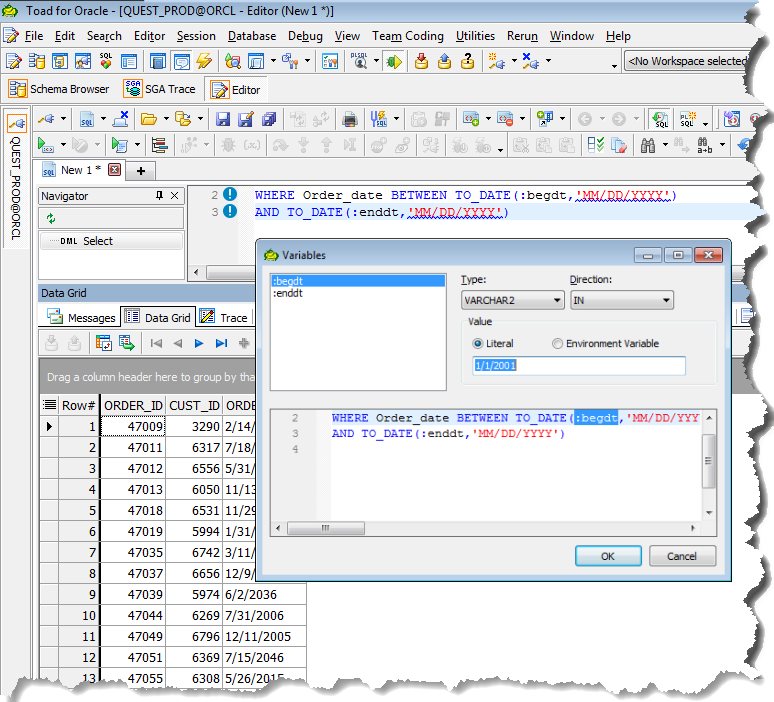# How do I JOIN result sets in a procedure

We have users that run their report queries in the Editor Window and export from the DATA GRID to Excel. The below is a sample and is working fine. We need to write this same process so that we can start using variables in the future. Keep in mind this is only a simple example to illustrate our goal, but IMGDATA and LMDATA are independent query result sets. We use IMGRPT1 to aggregate these to retain only active records from IMGDATA. Is it possible to rewrite this to give our users the ability to use variables - such as entering their date range in BegDate and EndDate?

Mike

Code:

--------------- C O M P I L E D A T A -----------------

WITH IMGDATA AS (SELECT
CLIENT_LOAN_NUMBER
FROM DITMGR.IMAGES
WHERE IMAGE_DATE BETWEEN TO_DATE('3/10/2019','MM/DD/YYYY')
AND TO_DATE('3/15/2019','MM/DD/YYYY')
),

`````` LMDATA AS (SELECT
LOAN_NUMBER,
CASE
WHEN LOAN_NUMBER IS NOT NULL THEN 'Y' ELSE 'N'
END AS LM
FROM MORTGMGR.MSP
WHERE STATUS_CODE = 'A'
),
``````

------------- A G G R E G A T E Q U E R I E S -------------

``````--Keep Active:

IMGRPT1 AS (SELECT A.*, B.LM
FROM IMGDATA A
LEFT JOIN (SELECT * FROM LMDATA) B
ON B.LOAN_NUMBER = A.CLIENT_LOAN_NUMBER
WHERE B.LM = 'A'
)
``````

-------------- G E N E R A T E R E P O R T ---------------

``SELECT * FROM IMGRPT1``

Can you not use bind variables?
e.g.

... ... ...
WHERE Order_date BETWEEN TO_DATE(:begin_dt,'MM/DD/YYYY')
AND TO_DATE(:end_dt,'MM/DD/YYYY')
... ... ...

Here's what happens:Thanks Gary for the reply. I need to use variables for our users to enter before running their query. The purpose is to minimize human error as much as possible. Thanks again for your reply.

Thanks Gary for the reply. Yes, bind variables will work fine. Thanks again!